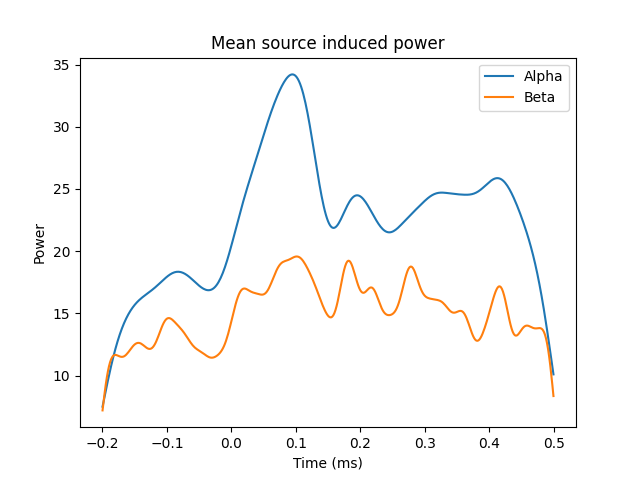# Compute induced power in the source space with dSPM#

Returns STC files ie source estimates of induced power for different bands in the source space. The inverse method is linear based on dSPM inverse operator.

```# Authors: Alexandre Gramfort <alexandre.gramfort@inria.fr>
#
```
```import matplotlib.pyplot as plt

import mne
from mne import io
from mne.datasets import sample

print(__doc__)
```

Set parameters

```data_path = sample.data_path()
meg_path = data_path / 'MEG' / 'sample'
raw_fname = meg_path / 'sample_audvis_raw.fif'
fname_inv = meg_path / 'sample_audvis-meg-oct-6-meg-inv.fif'
tmin, tmax, event_id = -0.2, 0.5, 1

# Setup for reading the raw data
events = mne.find_events(raw, stim_channel='STI 014')

include = []

picks = mne.pick_types(raw.info, meg=True, eeg=False, eog=True,

event_id = 1
events = events[:10]  # take 10 events to keep the computation time low
# Use linear detrend to reduce any edge artifacts
epochs = mne.Epochs(raw, events, event_id, tmin, tmax, picks=picks,

# Compute a source estimate per frequency band
bands = dict(alpha=[9, 11], beta=[18, 22])

stcs = source_band_induced_power(epochs, inverse_operator, bands, n_cycles=2,
use_fft=False, n_jobs=None)

for b, stc in stcs.items():
stc.save('induced_power_%s' % b, overwrite=True)
```
```Opening raw data file /home/circleci/mne_data/MNE-sample-data/MEG/sample/sample_audvis_raw.fif...
Read a total of 3 projection items:
PCA-v1 (1 x 102)  idle
PCA-v2 (1 x 102)  idle
PCA-v3 (1 x 102)  idle
Range : 25800 ... 192599 =     42.956 ...   320.670 secs
320 events found
Event IDs: [ 1  2  3  4  5 32]
Reading inverse operator decomposition from /home/circleci/mne_data/MNE-sample-data/MEG/sample/sample_audvis-meg-oct-6-meg-inv.fif...
[done]
[done]
305 x 305 full covariance (kind = 1) found.
Read a total of 4 projection items:
PCA-v1 (1 x 102) active
PCA-v2 (1 x 102) active
PCA-v3 (1 x 102) active
Average EEG reference (1 x 60) active
22494 x 22494 diagonal covariance (kind = 2) found.
22494 x 22494 diagonal covariance (kind = 6) found.
22494 x 22494 diagonal covariance (kind = 5) found.
Did not find the desired covariance matrix (kind = 3)
Computing patch statistics...
[done]
Computing patch statistics...
[done]
Read a total of 4 projection items:
PCA-v1 (1 x 102) active
PCA-v2 (1 x 102) active
PCA-v3 (1 x 102) active
Average EEG reference (1 x 60) active
Source spaces transformed to the inverse solution coordinate frame
2 matching events found
Setting baseline interval to [-0.19979521315838786, 0.0] sec
Applying baseline correction (mode: mean)
Created an SSP operator (subspace dimension = 3)
3 projection items activated
Preparing the inverse operator for use...
Scaled noise and source covariance from nave = 1 to nave = 1
Created the regularized inverter
Created an SSP operator (subspace dimension = 3)
Created the whitener using a noise covariance matrix with rank 302 (3 small eigenvalues omitted)
Computing noise-normalization factors (dSPM)...
[done]
Picked 305 channels from the data
Computing inverse...
Eigenleads need to be weighted ...
Reducing data rank 305 -> 302
Computing source power ...
[Parallel(n_jobs=1)]: Using backend SequentialBackend with 1 concurrent workers.
[Parallel(n_jobs=1)]: Done   1 out of   1 | elapsed:    2.4s remaining:    0.0s
[Parallel(n_jobs=1)]: Done   1 out of   1 | elapsed:    2.4s finished
[done]
[done]
Writing STC to disk...
[done]
Writing STC to disk...
[done]
```

plot mean power

```plt.plot(stcs['alpha'].times, stcs['alpha'].data.mean(axis=0), label='Alpha')
plt.plot(stcs['beta'].times, stcs['beta'].data.mean(axis=0), label='Beta')
plt.xlabel('Time (ms)')
plt.ylabel('Power')
plt.legend()
plt.title('Mean source induced power')
plt.show()
```Total running time of the script: ( 0 minutes 8.033 seconds)

Estimated memory usage: 587 MB

Gallery generated by Sphinx-Gallery